# Method of obtaining probability plot points

Probability Plot creates an estimated cumulative distribution function (CDF) from your sample by plotting the value of each observation (including repeated values) against its estimated cumulative probability.

Minitab calculates the estimated cumulative probability by using one of the following formulas, according to what is selected in File > Options > Individual Graphs > Probability Plots (the default is median rank). For each formula, let n equal the number of observations and i equal the rank-order of each observation such that i = 1 for the smallest value and i = n for the largest.

Median Rank (Benard)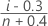Mean Rank (Herd-Johnson)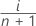Modified Kaplan-Meier (Hazen)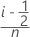Kaplan-Meier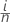###### Note

The Kaplan-Meier method results in p = 1 for the largest observation. Because the resulting value cannot be used in the plot, Minitab instead calculates the largest p as 90% of the distance between the prior p and 1.

The fitted distribution line represents the CDF for the selected theoretical distribution with the indicated parameters (either estimated or historical). If you do not provide historical parameters, Minitab estimates the parameters using least squares estimation (normal or lognormal distribution) or maximum likelihood estimation (other distributions).

The y-values (and in some cases the x-values) are transformed so that the fitted line is linear. Tick labels, however, remain consistent with the untransformed values. Thus, to the extent that the selected distribution fits your data, the plotted points form a straight line.

The following table shows the transformations used for each distribution.

Distribution X-coordinate Y-coordinate (score)
Normal data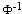(p)
Lognormal ln(data)(p)
3-parameter lognormal ln(data - threshold)(p)
Gamma ln(data) G-1(p), k
3-parameter gamma ln(data - threshold) G-1(p), k
Exponential ln(data) ln(-ln(1 - p))
2-parameter exponential ln(data - threshold) ln(-ln(1 - p))
Smallest extreme value data ln(-ln(1 - p))
Weibull ln(data) ln(-ln(1 - p))
3-parameter Weibull ln(data - threshold) ln(-ln(1 - p))
Largest extreme value data -ln(-ln(p))
Logistic data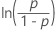Loglogistic ln(data)3-parameter loglogistic ln(data - threshold)###### Important

If you plot data unadjusted for threshold, distribution fit is not indicated by a straight line.

## Notation

TermDescription(p)value returned for p by the inverse CDF for the standard normal distribution.